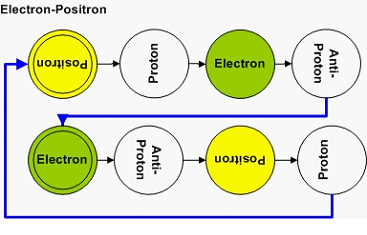# ARE Neutral Majorama PARTICLES 2^N NUMBERSARE Neutral Majorama PARTICLES 2^N NUMBERS

YES. I am sure the numerologists will have a field day..:)

As in any good Horoscope, there is a caveat. There are exclusions to the Prime Rule. They are associated with another nice mathematical formula, the formula for 2 to the power of another number. (2,4,8,16…)

TWO is the precursor to a Gamma photon from the annihilation of a Positron-Electron pair.FOUR is the Pion ZeroEIGTH is the KAON ZEROLater, I will show how the mathematical space topology and mathematical instrumentation needed to solve the Riemann Hypothesis also describes the mass of the Hyperons…:)

A hint. Look at the Fundamental Dilator coherence 'energy' diagram. The degenerated states on the two potential wells lose their degeneration due to the finite velocity of light, which creates a time delay for a spatial rotation within the 3D Lightspeed Expanding Hypersphere.

Think about what does it means to be real…:) and read the Meaning of Material Existence blog I wrote in the past.ARE THERE Neutral PARTICLES = 2*N NUMBERS

As in any good Horoscope, there is always something for everyone. It just happens that 2N is also a valid mapping for Neutral Particles.

Of course, since they decompose into opposite charged particles, they have to be the SUM OF TWO PRIMES (Goldbach's Conjecture) or 2 Particles supported by the Riemann Conjecture…:) . All together supported by the ABC Conjecture..:)

SIX is the DELTA ZERONow that you know the answer to the question, it should be easier to derive the equation that describes the process...:) I wrote a book called The Flying Orchestra, which represents our journey through the Universe inside the Ligthspeed Expanding Hyperspherical Universe. Most of us :) are composed of isotopes, which turned out to be quite harmonious dimensional notes. The Hyperons are the basic notes of our Universe, that is, the most unique, cacophonous notes one can create- like Heavy Metal...:)

In comparison, the isotopes are like Mozart Symphonies...NAH....in fact, they are quite boring and more like Kenny G

In the next few blogs, I will address the isotopes, the mass equation (or God Equation if you so prefer :) .

I am awaiting for Steven Chu to say something before I write about how to make a neutral particle accelerator or anything accelerator or dimensional accelerator...:)

Cheers,

MP
Currently unrated

### New Comment

required

required (not published)

optional

required